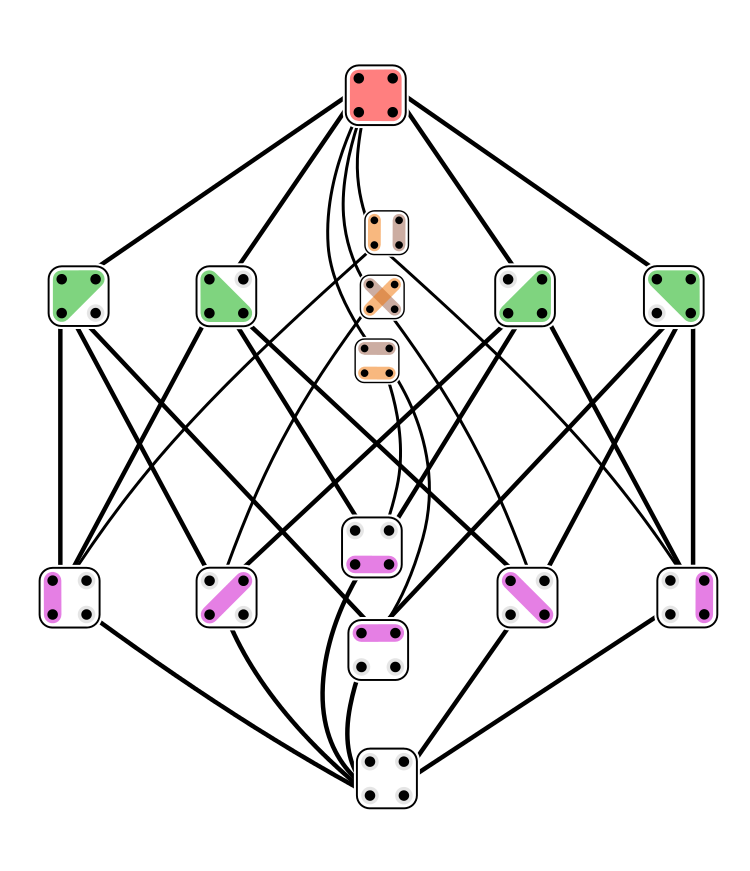Here are some hints. Spoiler alert: I'll do part 2 of this exercise.

A **[partition](https://en.wikipedia.org/wiki/Partition_of_a_set)** of a set is a way of writing it as a disjoint union of nonempty subsets. These subsets are called **blocks**. Here are all 52 partitions of a 5-element set, as drawn by [Watchduck](https://commons.wikimedia.org/wiki/File:Set_partitions_5;_circles.svg) on Wikicommons:We say one partition \\(P\\) of a set is **finer** than another partition \\(P'\\) if every block of \\(P'\\) is a union of blocks of \\(P\\). One could say "finer than or equal to", but people say just "finer". In this situation we also say \\(P'\\) is **coarser** than \\(P\\).

If \\(P\\) is finer than \\(P'\\), Fong and Spivak write \\(P \le P'\\) . This is a somewhat arbitrary choice: we could have done it the other way. This relation \\(\le\\) makes the collection of all partitions of a set \\(S\\) into a poset, which is usually called \\(\Pi(S)\\). This poset has a lot of interesting properties.

Here's a picture of all 15 partitions of a 4-element set, with finer ones nearer the bottom, and edges pointing from each partition to the next finer ones. This way of drawing a poset is called a [Hasse diagram](https://en.wikipedia.org/wiki/Hasse_diagram):The picture was drawn by [Tilman Piesk](https://commons.wikimedia.org/wiki/File:Set_partitions_4;_Hasse;_circles.svg) on Wikicommons. Click on it for a lot more information.

For any two partitions \\(P,P'\\) of a set \\(S\\), there is a unique finest partition \\(P \vee P'\\) that is coarser than both. In other words:

1. \\(P \le P \vee P'\\) and \\(P' \le P \vee P'\\).

2. If \\(Q\\) is any partition with \\(P \le Q\\) and \\(P' \le Q\\), then \\(P \vee P' \le Q\\).

This is called the **join** of \\(P\\) and \\(P'\\).

There's also a unique coarsest partition \\(P \wedge P'\\) that is finer than both \\(P\\) and \\(P'\\). This is called the **meet** of \\(P\\) and \\(P'\\).